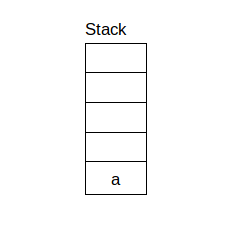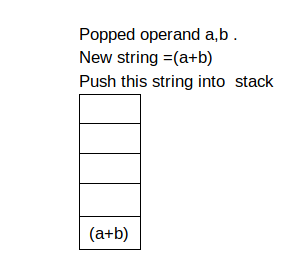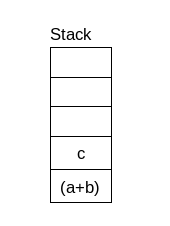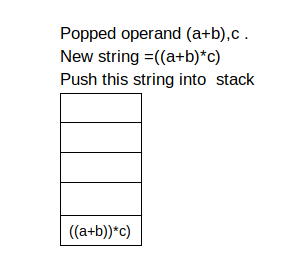×

Search anything:

# Converting Postfix to Infix using Stack

#### Algorithms Data Structures Stack postfix expression infix expressionReading time: 20 minutes | Coding time: 10 minutes

We have explored an algorithm to convert a Postfix expression to Infix expression using Stack.

• Postfix
• If operator appear before operand in the expression then expression is known as Postfix operation.
• Infix
• If operator is in between every pair of operands in the expression then expression is known as Infix operation.
• Postfix Expression can easily solve by machine(computers) but for human postfix operation is difficult to evaluate.
• in the back-end side(machine) Postfix expression is necessary and in the front-end side (user) Infix expression is necessary.
• So let's Study how to convert Postfix expression into infix expression.
• Prerequisite :
1. Basics of Stack data structure
2. Basics of List in Python

## Steps to Convert Postfix to Infix :

1. Read the symbol from the input .based on the input symbol go to step 2 or 3.
2. If symbol is operand then push it into stack.
3. If symbol is operator then pop top 2 values from the stack.
4. this 2 popped value is our operand .
5. create a new string and put the operator between this operand in string.
6. push this string into stack.
7. At the end only one value remain in stack which is our infix expression.
• Example :
• Input postfix expression is ab+c*
• Initial empty stack
•• first input(character) is operand so pushed into stack.
•• Second character is also operand so pushed into stack.
•• Third character is operator so we need to popped 2 value from stack.then we need to add operator between this two operand.
•• fourth character is operand so we need to push into stack.
•• fifth character is operator so we need to popped 2 value from stack.then we need to add operator between this two operand.
•## Implementation :

``````def getInfix(str):
stack=[]
for j in range(len(str)) :
if operand(str[j]) :
stack.append(str[j])
else :
operator1=stack.pop()
operator2=stack.pop()
stack.append("(" + operator2 + str[j] + operator1 + ")")
return stack.pop()
def operand(char) :
if (char &gt= 'a' and char &lt= 'z') or (char &gt= 'A' and char &lt= 'Z') :
return True
else :
return False
str=['a','b','*','c','+','d','*','e','/']
print(getInfix(str))
``````

## Output :

``````((((a*b)+c)*d)/e)
``````

## Time Complexity :

Time complexity of above implementation is O(n) where n is number of character in postfix expression.#### Akshay Gopani

Intern at OpenGenus (2019) | Bachelor of Technology (2017 to 2021) in Information and Communication Technology at Ahmedabad University

Converting Postfix to Infix using Stack